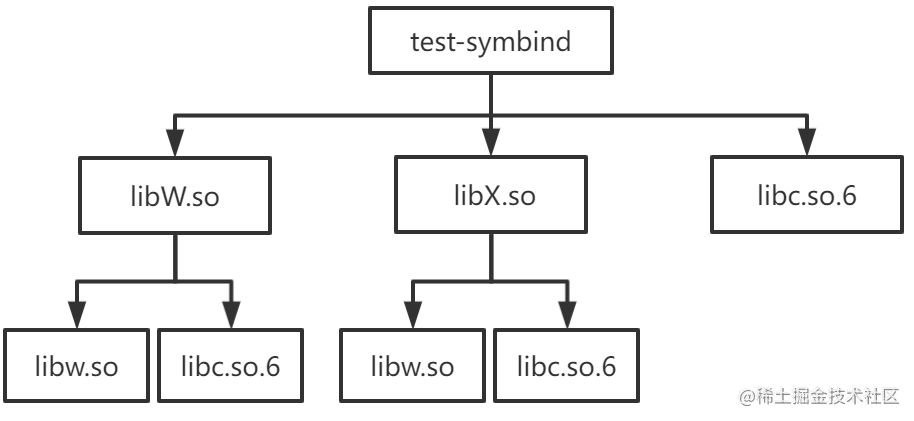# 深入淺出 Sanitizer Interceptor 機制

## Sanitizer 簡介

Sanitizer 是由 Google 開源的一系列動態代碼分析工具，從 Clang 3.1 和 GCC 4.8 開始被集成在 Clang 和 GCC 中，能夠幫助程序員快速準確地在運行時定位程序中的內存錯誤和多線程錯誤。Sanitizer 工具集包括：

• LeakSanitizer (LSan)：用於檢測內存泄漏
• UndefinedBehaviorSanitizer (UBSsan)：用於檢測未定義行為
• MemorySanitizer (MSan)：用於檢測未初始化內存的訪問

• ASan 編譯時會在每一處內存讀寫語句之前插入代碼，根據每一次訪問的內存所對應的影子內存 ( shadow memory，就是使用額外的內存來記錄常規的內存狀態）的狀態來檢測本次內存訪問是否合法。還會在棧變量和全局變量附近申請額外內存作為危險區，用於檢測內存溢出。
• ASan 運行時庫會替換 malloc/free, operator new/delete 等內存分配函數的實現，這樣應用程序的內存分配都由 ASan 實現的內存分配器負責。ASan 內存分配器會在它分配的堆內存附近申請額外內存用於檢測堆內存溢出，還會將被釋放的內存優先放在隔離區 (quarantine) 用於檢測像 heap-use-after-free 這樣的堆內存錯誤。

## Symbol interposition

1. 一個最簡單的方式就是在我們的應用程序中定義一個同名的 malloc 函數
2. 還有一種方式就是將我們的 malloc 函數實現在 libmymalloc.so 中，然後在運行我們的應用程序之前設置環境變量 `LD_PRELOAD=/path/to/libmymalloc.so`

When resolving symbolic references, the dynamic linker examines the symbol tables with a breadth-first search. That is, it first looks at the symbol table of the executable program itself, then at the symbol tables of the `DT_NEEDED` entries (in order), and then at the second level `DT_NEEDED` entries, and so on.

``````\$ cat main.c
extern int W(), X();

int main() { return (W() + X()); }

\$ cat W.c
extern int b();

int a() { return (1); }
int W() { return (a() - b()); }

\$ cat w.c
int b() { return (2); }

\$ cat X.c
extern int b();

int a() { return (3); }
int X() { return (a() - b()); }

\$ cat x.c
int b() { return (4); }

\$ gcc -o libw.so -shared w.c
\$ gcc -o libW.so -shared W.c -L. -lw -Wl,-rpath=.
\$ gcc -o libx.so -shared x.c
\$ gcc -o libX.so -shared X.c -L. -lx -Wl,-rpath=.
\$ gcc -o test-symbind main.c -L. -lW -lX -Wl,-rpath=.

````````````\$ LD_DEBUG="symbols:bindings" ./test-symbind
1884890:        symbol=a;  lookup in file=./test-symbind 
1884890:        symbol=a;  lookup in file=./libW.so 
1884890:        binding file ./libW.so  to ./libW.so : normal symbol `a'
1884890:        symbol=b;  lookup in file=./test-symbind 
1884890:        symbol=b;  lookup in file=./libW.so 
1884890:        symbol=b;  lookup in file=./libX.so 
1884890:        symbol=b;  lookup in file=/lib/x86_64-linux-gnu/libc.so.6 
1884890:        symbol=b;  lookup in file=./libw.so 
1884890:        binding file ./libW.so  to ./libw.so : normal symbol `b'
1884890:        symbol=a;  lookup in file=./test-symbind 
1884890:        symbol=a;  lookup in file=./libW.so 
1884890:        binding file ./libX.so  to ./libW.so : normal symbol `a'
1884890:        symbol=b;  lookup in file=./test-symbind 
1884890:        symbol=b;  lookup in file=./libW.so 
1884890:        symbol=b;  lookup in file=./libX.so 
1884890:        symbol=b;  lookup in file=/lib/x86_64-linux-gnu/libc.so.6 
1884890:        symbol=b;  lookup in file=./libw.so 
1884890:        binding file ./libX.so  to ./libw.so : normal symbol `b'

``````
• 函數 a 在 libW.solibX.so 中都有一份定義，但因為是按照 test-symbind, libW.so, libX.so, libc.so.6, libw.so, libx.so 的順序查找符號定義的，所以最終所有對函數 a 的引用都綁定到 libW.so 中函數 a 的實現
• 函數 b 在 libw.solibx.so 中都有一份定義，但因為是按照 test-symbind, libW.so, libX.so, libc.so.6, libw.so, libx.so 的順序查找符號定義的，所以最終所有對函數 b 的引用都綁定到 libw.so 中函數 b 的實現

• 方式一：在我們的應用程序中定義一個同名的 malloc 函數。動態鏈接器在查找符號時 executable 的順序在 libc.so.6 之前，因此所有對 malloc 的引用都會綁定到 executable 中 malloc 的實現。
• 方式二：將我們的 malloc 函數實現在 libmymalloc.so 中，然後在運行我們的應用程序之前設置環境變量 LD_PRELOAD=/path/to/libmymalloc.so。動態鏈接器在查找符號時 libmymalloc.so 的順序在 libc.so.6 之前，因此所有對 malloc 的引用都會綁定到 libmymalloc.so 中 malloc 的實現。

``````// test.cpp
#include <iostream>
int main() {
}

``````

``````\$ objdump -p test-gcc-asan | grep NEEDED
NEEDED               libasan.so.5
NEEDED               libstdc++.so.6
NEEDED               libm.so.6
NEEDED               libgcc_s.so.1
NEEDED               libc.so.6

``````

``````\$ LD_DEBUG="bindings" ./test-gcc-asan
3309213:        binding file /lib/x86_64-linux-gnu/libc.so.6  to /usr/lib/x86_64-linux-gnu/libasan.so.5 : normal symbol `malloc' [GLIBC_2.2.5]
3309213:        binding file /lib64/ld-linux-x86-64.so.2  to /usr/lib/x86_64-linux-gnu/libasan.so.5 : normal symbol `malloc' [GLIBC_2.2.5]
3309213:        binding file /usr/lib/x86_64-linux-gnu/libstdc++.so.6  to /usr/lib/x86_64-linux-gnu/libasan.so.5 : normal symbol `malloc' [GLIBC_2.2.5]

``````

## Sanitizer interceptor

Sanitizer interceptor 存在一個測試文件interception_linux_test.cpp

``````#include "interception/interception.h"
#include "gtest/gtest.h"

static int InterceptorFunctionCalled;

DECLARE_REAL(int, isdigit, int);

INTERCEPTOR(int, isdigit, int d) {
++InterceptorFunctionCalled;
return d >= '0' && d <= '9';
}

namespace __interception {

TEST(Interception, Basic) {
EXPECT_TRUE(INTERCEPT_FUNCTION(isdigit));

// After interception, the counter should be incremented.
InterceptorFunctionCalled = 0;
EXPECT_NE(0, isdigit('1'));
EXPECT_EQ(1, InterceptorFunctionCalled);
EXPECT_EQ(0, isdigit('a'));
EXPECT_EQ(2, InterceptorFunctionCalled);

// Calling the REAL function should not affect the counter.
InterceptorFunctionCalled = 0;
EXPECT_NE(0, REAL(isdigit)('1'));
EXPECT_EQ(0, REAL(isdigit)('a'));
EXPECT_EQ(0, InterceptorFunctionCalled);
}

}  // namespace __interception

``````

• `INTERCEPTOR(int, isdigit, int d) { ... }` 用於將函數 `isdigit` 的實現替換為 { ... } 的實現
• 在代碼中調用 `isdigit` 之前，需要先調用 `INTERCEPT_FUNCTION(isdigit)`。如果 `INTERCEPT_FUNCTION(isdigit)` 返回為 true，則説明成功替換了將 libc 中 `isdigit` 函數的實現。
• `REAL(isdigit)('1')` 用於調用真正的 `isdigit` 實現，不過在調用 `REAL(isdigit)('1')` 之前需要先 `DECLARE_REAL(int, isdigit, int)`

``````INTERCEPTOR(int, isdigit, int d) {
++InterceptorFunctionCalled;
return d >= '0' && d <= '9';
}

INTERCEPT_FUNCTION(isdigit);

DECLARE_REAL(int, isdigit, int);
REAL(isdigit)('1');

``````
• 我們首先看下 INTERCEPTOR 宏做了哪些事情

• 首先在 __interception namespace 中定義了一個函數指針 real_isdigit，該函數指針實際上在 INTERCEPT_FUNCTION 宏中會被設置為指向真正的 `isdigit` 函數地址。
• 然後將 `isdigit` 函數設置為弱符號 (weak)，並且將 `isdigit` 設置成 `__interceptor_isdigit` 的別名 (alias)。
• 最後將我們自己版本的 `isdigit` 函數邏輯實現在 `__interceptor_isdigit` 函數中

``````extern "C" int __interceptor_isdigit(int d);
extern "C" int isdigit(int d) {
fprintf(stderr, "my_isdigit_interceptor\n");
return __interceptor_isdigit(d);
}

``````

• 接着我們看下 INTERCEPT_FUNCTION 宏做了哪些事情

• INTERCEPT_FUNCTION 宏展開後就是對 __interception::InterceptFunction 函數的調用。`InterceptFunction`函數定義
``````namespace __interception {
// If the lookup using RTLD_NEXT failed, the sanitizer 運行時庫 is
// later in the library search order than the DSO that we are trying to
// intercept, which means that we cannot intercept this function. We still
// want the address of the real definition, though, so look it up using
// RTLD_DEFAULT.

// In case `name' is not loaded, dlsym ends up finding the actual wrapper.
// We don't want to intercept the wrapper and have it point to itself.
}
}

bool InterceptFunction(const char *name, uptr *ptr_to_real, uptr func,
uptr wrapper) {
return addr && (func == wrapper);
}
}  // namespace __interception

``````

• dlsym 的第一個參數為 RTLD_DEFAULT 時，查找名為 name 的函數地址的順序就是前面提到的 executable, preload0.so, preload1.so needed0.so, needed1.so, needed2.so, needed0_of_needed0.so, needed1_of_needed0.so, ... 這個順序。

• dlsym 的第一個參數為 RTLD_NEXT 時，則是以當前 object 後面動態庫為起點去查找名為 name 的函數的地址

• 最後我們看下 DECLARE_REAL 宏 和 REAL 宏做了哪些事情

DECLARE_REAL 展開後就是聲明瞭在 __interception namespace 中存在一個指向被替換函數真正實現的函數指針，REAL 宏就是通過這個函數指針來調用被替換函數的真正實現。

## 總結

ASan 基於 sanitizer interceptor 機制替換了 malloc/free 這類的內存分配/釋放函數，使得所有的內存分配和釋放都由 ASan 實現的內存分配器負責，這樣 ASan 就能很容易檢測到 heap-use-after-free，double-free 這樣的堆內存錯誤。

## 直播預告